• 使用目标检测中流行的SSD算法，智能识别中国马路上的交通标志牌。文档末端附开源代码连接
• 交通标牌检测与识别

千次阅读 多人点赞 2017-03-09 19:39:27
关于交通标牌检测的博客和论文非常多，例如，本人最近在博客上就看到有一篇简单的交通标志检测与识别介绍文章《自动驾驶之眼——摄像头是如何认识交通标志的？》，该文很简洁明了地阐述了交通标志检测识别的主要流程...

关于交通标牌检测的博客和论文非常多，例如，本人最近在博客上就看到有一篇简单的交通标志检测与识别介绍文章《自动驾驶之眼——摄像头是如何认识交通标志的？》，该文很简洁明了地阐述了交通标志检测识别的主要流程。本文将结合上学期的课程设计，来整理一下交通标识牌检测与识别的思路与实现方法。

一、要求

首先要明确一下本文到底是要干什么。本文要完成基于视觉的交通标识牌检测与识别，说白了，就两个事：1）在一张图中找到交通标识牌在哪里（检测）；2）认清楚这个标识牌是啥，表达的什么意思（识别）。那么最后得到的结果预览如下：二、使用数据

交通标识牌种类数不胜数，我国的交通标志一共有一百余种，按类别可分为黄底黑边的警告标志、白底红圈的禁令标志、蓝底白字的指示标志，形状上以三角形、圆形和矩形为主。本文主要是为了介绍一下交通标识牌的识别流程和一些主要方法的实现，为了简化工作，本文挑选了以下五类交通标识牌。可以看出来，博主用心良苦，选择的交通标识牌具有很清楚的特征：1）颜色上，这五类交通标识牌的外边框都是红色的；2）形状上，标识牌都是标准的圆形。这事实上也表明了，交通标识牌具有着鲜明的特征，故无论是人眼还是机器，都较易识别。(其他种类的交通标志牌也是一样，利用形状和颜色特征来处理)

三、使用方法
在我看来，目前处理交通标牌识别的主要有两种方法，1）传统的图像处理+机器学习办法；2）最近很火的深度学习。那么本文采用的是前者，后者后续再进行介绍。

1>检测：颜色和形状。

交通标志牌为了起到其警示作用，在颜色和形状上都有着易区分性，如本文所讨论的五类标志牌，颜色特征为外框均为鲜艳的红色；形状特征为均为圆形。于是，检测的思路如下,最终得到了圆形部分的交通标牌：接下来，主要分为颜色分割和形状检测两部分进行讨论：

基于颜色分割的图像二值化处理：

最直观、简单的是利用RGB颜色空间来描述图像的色彩情况，但是，RGB色彩空间极易受到光线情况的影响，鲁棒性并不是很好，所以在相关论文中，你会发现，很少有人直接使用RGB色彩空间进行色彩分割。而实际上，本人拿有限的样本和测试集进行测试，RGB色彩分割效果在图像成像质量较理想的时候效果极佳，但是的确容易受到干扰。本文此处选择了HSI色彩空间模型进行色彩分割。先来点理论知识：

色调H（Hue）：与光波的波长有关，它表示人的感官对不同颜色的感受，如红色、绿色、蓝色等，它也可表示一定范围的颜色，如暖色、冷色等。

饱和度S（Saturation）：表示颜色的纯度，纯光谱色是完全饱和的，加入白光会稀释饱和度。饱和度越大，颜色看起来就会越鲜艳，反之亦然。

亮度I（Intensity）：对应成像亮度和图像灰度，是颜色的明亮程度。

从理论上看，HSI色彩空间将饱和度和亮度信息独立了出来，这样一定程度上就降低了光线带来的影响。听上去很有道理，但是，实际上，这也仅仅是一定程度上降低了亮度和色彩的耦合关系，并不是完全地进行了解耦，所以，效果会有提升，但是很难带来质的改变（这是笔者自己的体验，也许是笔者能力不足，实现得不是很理想

那么从RGB色彩空间转换到HSI空间的转换公式如下：函数RGB2HSI是将RGB色彩空间转换到HSI色彩空间，其转换的过程参照式(2.2)，最后将饱和度S和强度I均放大100倍，便于操作。 最后得到的H、 S、 I的取值范围分别为[0,360]、 [0,100]、 [0,100]。

void RGB2HSV(double red, double green, double blue, double& hue, double& saturation,
double& intensity )
{
double r,g,b;
double h,s,i;
double sum;
double minRGB,maxRGB;
double theta;
r = red/255.0;
g = green/255.0;
b = blue/255.0;
minRGB = ((r<g)?(r):(g));
minRGB = (minRGB<b)?(minRGB):(b);
maxRGB = ((r>g)?(r):(g));
maxRGB = (maxRGB>b)?(maxRGB):(b);
sum = r+g+b;
i = sum/3.0;
if( i<0.001 || maxRGB-minRGB<0.001 )
{
h=0.0;
s=0.0;
}
else
{
s = 1.0-3.0*minRGB/sum;
theta = sqrt((r-g)*(r-g)+(r-b)*(g-b));
theta = acos((r-g+r-b)*0.5/theta);
if(b<=g)
h = theta;
else
h = 2*PI - theta;
if(s<=0.01)
h=0;
}
hue = (int)(h*180/PI);
saturation = (int)(s*100);
intensity = (int)(i*100);
}
在得到HSI空间的基础上，分割出红色像素，事实上这个阈值最好时自己调出来，无论是基于哪个色彩空间，网上的代码或者论文中的数值都是个参考，自己调出来的才靠谱嘛，代码如下：
//得到图像参数
int width = src.cols; //图像宽度
int height = src.rows; //图像高度
//色彩分割
double B=0.0,G=0.0,R=0.0,H=0.0,S=0.0,I=0.0;
Mat Mat_rgb = Mat::zeros( src.size(), CV_8UC1 );
int x,y,px,py; //循环
for (y=0; y<height; y++)
{
for ( x=0; x<width; x++)
{
// 获取 BGR 值
B = src.at<Vec3b>(y,x);
G = src.at<Vec3b>(y,x);
R = src.at<Vec3b>(y,x);
RGB2HSV(R,G,B,H,S,I);
//红色：337-360
if((H>=337 && H<=360||H>=0&&H<=10)&&
S>=12&&S<=100&&V>20&&V<99)
{
Mat_rgb.at<uchar>(y,x) = 255; //分割出红色
}
}
}
分割效果可见如下组图，由近至远：⚠️⚠️⚠️ 注意：有一个很严肃的问题我这里没有提，那就是图像预处理！做图像处理的很重要的一个步骤就是图像预处理，预处理做好了，后面的问题复杂度也就降低了许多。实际上，用颜色分割来二值化图像也可以看作一种预处理。那么颜色分割之前有不有必要做图像预处理呢？是有的。举个例子，我那我的MATE8在学校里拍了一张照，然后使用手机相机自带的功能，调整其色彩饱和度，亮度等，得到以下两种图片：相机拍的原图                                                  手机调整饱和度、亮度后
这两种图片，显然右边的将更有利于颜色分割！（不信可以试试哦）。本文主要以介绍交通标牌的主要流程为主，预处理的方法包括直方图均衡化、白平衡、亮度调节等等这些就不仔细纠结了，但是，不代表这部分不重要，图像预处理往往一定程度上决定了最后的效果。

基于形状（圆形）检测的ROI提取
在进行颜色分割之后，得到的只是一个粗略的交通标志牌ROI区域， 还会留下一些噪声以及一些和目标区域面积相当或者比目标面积略大的区域，这时候就还需要进行一些图像预处理，为准确检测交通标志牌打下坚实基础。由于交通标志最明显的特征是其颜色和形状，在用颜色分割之后，我们可以通过形状特征来去除其余的干扰。对于本文的研究对象而言，交通标志牌的形状为圆形，可以采用经典的Hough变换进行圆检测，该方法准确性高，但是计算量大，耗时且占用较大内存；也可以采用圆度的方法来提取圆形，该方法原理简单，计算量小，准确率高。综合考虑，本文使用基于圆度的圆检测算法。大概流程如下，后文还会详细介绍：图有点不太清楚，下文中对于关键的部分会再次给出效果图。
中值滤波，这个没啥好说的，图上效果不是很明显，但是实际上可以一定程度上滤掉单个噪点，对得到准确的结果会有一定的帮助；

形态学处理，最后我们的目的是要得到一个封闭的区域，所以，颜色分割后的结果很可能不会是比较理想封闭的圆形，那么选用的3×3腐蚀模板，7×7膨胀模板，这样检测到的圆形将基本不会产生缺口，保证是一个封闭的形状。图像填充，有了上述步骤得到的封闭圆形，我们接下来就可以填充封闭图形了（这里你可能会问，为啥要这样做。实际上直接进行Hough圆检测可以得到ROI结果，但是本文是换了一个思路，使用圆度来判断圆形，所以算法需要一个实心区域），代码如下：
void fillHole(const Mat srcBw, Mat &dstBw)
{
Size m_Size = srcBw.size();
Mat Temp=Mat::zeros(m_Size.height+2,m_Size.width+2,srcBw.type());//延展图像
srcBw.copyTo(Temp(Range(1, m_Size.height + 1), Range(1, m_Size.width + 1)));
cv::floodFill(Temp, Point(0, 0), Scalar(255));//填充区域
Mat cutImg;//裁剪延展的图像
Temp(Range(1, m_Size.height + 1), Range(1, m_Size.width + 1)).copyTo(cutImg);
dstBw = srcBw | (~cutImg);
}轮廓检测，初步筛选ROI，要想使用基于圆度的圆检测算法，则需要从图像中提取初步的ROI来进行筛选。这里使用轮廓检测法来检测图片中的ROI区域。可以看到，一些细小的噪声也被检测进来。所以，本文先通过对检测区域的宽高比、面积大小进行限制，筛选出有效的检测区域，经过实验，可以确定宽高比限制在0.5-2之间，面积最小值设定为400，可以进一步得到下图的检测效果，可以看到，此时小面积的噪声已经被排除。代码如下：
//找轮廓
vector<vector<Point> > contours;
vector<Vec4i> hierarchy;
findContours( Mat_rgb, contours, hierarchy, CV_RETR_EXTERNAL,
CV_CHAIN_APPROX_SIMPLE, Point(0, 0) );
/// 多边形逼近轮廓 + 获取矩形和圆形边界框
vector<vector<Point> > contours_poly( contours.size() );
vector<Rect> boundRect( contours.size() );
vector<Point2f>center( contours.size() );
//得到轮廓矩形框
for( int i = 0; i < contours.size(); i++ )
{
approxPolyDP( Mat(contours[i]), contours_poly[i], 3, true );
boundRect[i] = boundingRect( Mat(contours_poly[i]) );
}
/// 画多边形轮廓 + 包围的矩形框
Mat drawing = Mat::zeros( Mat_rgb.size(), CV_8UC3 );
for( int i = 0; i< contours.size(); i++ )
{
Rect rect = boundRect[i];
//首先进行一定的限制，筛选出区域
//高宽比限制
float ratio = (float)rect.width / (float)rect.height;
//轮廓面积
float Area = (float)rect.width * (float)rect.height;
float dConArea = (float)contourArea(contours[i]);
float dConLen = (float)arcLength(contours[i],1);
if(dConArea <400)//ROI 区域面积限制
continue;
if(ratio>2||ratio<0.5)//ROI 区域宽高比限制
continue;
//检测到了！
Scalar color = Scalar( rng.uniform(0, 255), rng.uniform(0,255), rng.uniform(0,255) );
//绘制轮廓和检测到的轮廓外接矩形
drawContours( drawing, contours_poly, i, color, 1, 8, vector<Vec4i>(), 0, Point() );
rectangle( drawing, boundRect[i].tl(), boundRect[i].br(), color, 2, 8, 0 );
rectangle( src, boundRect[i].tl(), boundRect[i].br(), color, 2, 8, 0 );
}

圆度算法检测，实际上这是利用了非常简单的数学约束，来对检测到的区域进行圆形验证。圆度定义如下：其中，S为圆的面积，L为圆的周长，C为圆度。圆度值越接近1，则表示该图形与圆形的契合程度越高。经过大量的实验，可以得出圆度大于0.5时，即 4 . 0  C 时，可以筛选出巨大部分的圆形。

ROI区域无效像素面积约束，这是进一步确定筛选后的ROI区域是目标圆形区域。该约束条件是基于ROI区域中圆形的缺失面积而得到的。前文中得到的ROI区域是包含圆形交通标志牌的矩形区域，如下图所示，可以将整个ROI区域分成1、2、3、4四块，其中红色部分为交通标志牌，灰色部分为ROI区域中的无效像素。可以直观地看到，1、2、3、4四块的无效像素满足一定的数学关系，有如下约束：圆度代码即为一个约束条件，对面个轮廓检测得到的ROI进行验证，无效像素面积约束则代码如下：
bool isCircle(const Mat srcBw,Mat& mytemp)//（待改进）
{//输入的是一个灰度图像
Mat temp = Mat::zeros( srcBw.size(), CV_8UC1 );;
bool iscircle = false;
//获得 srcBw 信息
int w = srcBw.cols;
int h = srcBw.rows;
int count1=0;//各部分的缺失像素计数器
int count2=0;
int count3=0;
int count4=0;
//将 srcBw 平均分成四份,进行访问缺失的像素个数、所占比重
//先访问左上
for(int i = 0;i < h/2;i ++)
{
for(int j = 0;j < w/2;j ++)
{
if(srcBw.at<uchar>(i,j) == 0)
{
temp.at<uchar>(i,j) = 255;
mytemp.at<uchar>(i,j) = 255;
count1 ++;
}
}
}
//右上
for(int i = 0;i < h/2;i ++)
{
for(int j = w/2-1;j < w;j ++)
{
if(srcBw.at<uchar>(i,j) == 0)
{
temp.at<uchar>(i,j) = 255;
mytemp.at<uchar>(i,j) = 255;
count2 ++;
}
}
}
//左下
for(int i = h/2-1;i < h;i ++)
{
for(int j = 0;j < w/2;j ++)
{
if(srcBw.at<uchar>(i,j) == 0)
{
temp.at<uchar>(i,j) = 255;
mytemp.at<uchar>(i,j) = 255;
count3 ++;
}
}
}
//右下
for(int i = h/2-1;i < h;i ++)
{
for(int j = w/2-1;j < w;j ++)
{
if(srcBw.at<uchar>(i,j) == 0)
{
temp.at<uchar>(i,j) = 255;
mytemp.at<uchar>(i,j) = 255;
count4 ++;
}
}
}
float c1 = (float)count1/(float)(w*h);//左上
float c2 = (float)count2/(float)(w*h);//右上
float c3 = (float)count3/(float)(w*h);//左下
float c4 = (float)count4/(float)(w*h);//右下
cout << "result: " << c1 << "," << c2
<< "," << c3 << "," << c4 << endl;
//限定每个比率的差值范围
if((c1>0.037&&c1<0.12)&&(c2>0.037&&c2<0.12)&&(c2>0.037&&c2<0.12)&&(c2>0.037
&&c2<0.12))
{
//限制差值,差值比较容错，相邻块之间差值相近，如左上=右上&&左下=右下或左上=左下&&右上=右下
if((abs(c1-c2)<0.04&&abs(c3-c4)<0.04)||(abs(c1-c3)<0.04&&abs(c2-c4)<0.04))
{
iscircle = true;
}
}
return iscircle;
}
利用轮廓检测、圆度约束和无效面积约束，可以测试得到如下效果图，最后，目标区域提取的效果如下：2>识别：SVM分类。
有了上文提取ROI的基础，分类过程实际上和我之前写过的箭头分类如出一辙。
图像预处理，首先将无效像素全部去除，只留下圆形ROI有效区域，然后进行二值化处理，二值化后的图像特征更为清晰可以选择所有像素作为特征，当然更科学的是Hu不变矩、Zernike不变矩、二者混合矩等特征。关于Hu、Zernike特征的代码网上比比皆是，这里仅推荐一个作为参考。本文为了简单实现框架，拿全部像素特征进行训练。准备好样本和测试集，并给这五类交通标牌设置标签“stop”，“20t”，“car forbidden”，“5”，“stop2”
SVM代码框架如下。
SVM训练
//*********************SVM 训练部分***********************
//准备开始训练
CvSVM classifier;
CvSVMParams SVM_params;
SVM_params.kernel_type = CvSVM::LINEAR; //使用 RBF 分类非线性问题
SVM_params.svm_type = CvSVM::C_SVC;
SVM_params.degree = 0;
SVM_params.gamma = 0.01;
SVM_params.term_crit = cvTermCriteria(CV_TERMCRIT_ITER, 1000, FLT_EPSILON);
SVM_params.C = 100;
SVM_params.coef0 = 0;
SVM_params.nu = 0;
SVM_params.p = 0.005;
classifier.train(train,labels ,Mat(),Mat(),SVM_params); //SVM 训练，线性核上述参数 C 起作用
SVM保存
classifier.save("model180.txt");
SVM读取
//这里载入分类器，方便直接训练
CvSVM classifier;
SVM预测
for (int i = 0;i < testdata.size() ; ++i)
{
int result = (int)classifier.predict(testdata[i]);
std::cout<<"测试样本"<<i+1<<"的测试结果为："
<<result<< " " << labelname[result-1] << "\n";
}
最后得到了文中开始展示的效果。
本文完整代码和数据，已托管在Github上https://github.com/lps683/TrafficsSignDetection。这些东西也许在高手看来不值一提，但是，若能给一部分人带来一些哪怕一点点收获，那么花这么多功夫写这篇文章也不算白费。
展开全文• 能够识别出不同场景环境下图片中的交通限速标志，将限速标志及其里面的数字分别标志出来，具有较强的鲁棒性。
• 龙源期刊网http://www.qikan.com.cn利用图像处理技术识别道路交通标志牌作者：施易廷来源：《电子技术与软件工程》2018年第02期摘要随着社会的发展，汽车的普及，现有的道路通过能力已经很难满足交通量快速增长的...

龙源期刊网

http://www.qikan.com.cn

利用图像处理技术识别道路交通标志牌

作者：施易廷

来源：《电子技术与软件工程》

2018

年第

02

随着社会的发展，汽车的普及，现有的道路通过能力已经很难满足交通量快速增长

的需要，因此导致了交通拥堵、事故频发等一系列问题。在这一背景下，智能交通（

ITS

）应

运而生。

ITS

是一个集通信，控制，检测和计算机等技术为一体的综合信息管理系统，而数字

图像处理则是

ITS

系统中一个重要的组成部分。本文主要介绍了如何利用交通标志牌其颜色的

特征，将其从自然环境图片中分离并且识别的过程。可以识别出缩放，偏斜等形式的交通标志

牌。

【关键词】图像处理

特征匹配

HSV

色彩空间

1

应用背景

自上世纪八十年代以来，我国的经济迅速发展，城市化的不断推进。汽车大范围普及，除

了公交车和出租汽车，小轿车也走进千家万户。虽然汽车的出现使人们的出行更加便捷，但是

随着汽车的大量增长，导致交通的负荷能力已经不能满足交通量的需求，出现了许多拥堵现

象，交通事故。为了能够更好地解决目前交通存在的各种问题，结合了计算机技术，电子信息

技术，通信技术等新兴技术的

ITS

诞生了。

ITS

可以有效地利用现有的交通设施、减少交通负

荷和环境污染，同时能够保证交通安全，提高运输效率，目前收到了各国的重视。

在智能交通领域中，车载视觉系统一直是一个重要的研究课题，也是目前没有解决的难题

之一，其在辅助驾驶方面具有重要的意义。交通标志牌在交通道路中不可或缺，起到提示方

向，指示操作的作用。能够实时的判断前方的交通标志牌，能够为司机提供很好的驾驶体验，

并且保障出行的安全。本文就将研究，介绍对交通标志牌识别的过程。

2

交通标志牌分类

我国的交通标志牌按功能类型区分可以分为主标志牌和辅助标志牌。

主标志牌又分为警告标志牌，禁令标志牌，指示标志牌，指路标志牌，旅游区标志牌，道

路施工安全标志牌和限速标志牌。而辅助标志牌多附设在主标志牌下，起到辅助说明的作用。

按照标志牌主要颜色分类可以分为：红色标志牌，蓝色标志牌，绿色标志牌，棕色标志

牌，黑色标志牌，白色标志牌，橙色或荧光橙色标志牌和荧光黄绿色标志牌。

按照形状分类可以分为：正等边三角形，圆形，倒等边三角形，八角形，叉形，方形等。

展开全文• yolov3是优秀的目标检测算法，笔者曾用yolov3完成《数字图像处理》的课程设计，课程设计是大型交通标志牌的检测，使用yolov3模型能够获得很好的效果，文件包括yolov3的论文、笔者录制的视频目标检测结果
• 现有基于低动态范围(LDR)图像的识别方法在良好的曝光环境下, 能取得较为理想的结果, 但其容易受照明条件的限制以及天气状况的影响, 稳健性不强。...实验结果表明, 该方法可较好地提高交通标志牌的检测与识别正确率。
•研究论文
• 行业资料-交通装置-一种宽视野交通标志牌.zip
• 行业资料-交通装置-一种LED交通标志牌系统.exe
• 行业资料-交通装置-一种低风荷载的交通标志牌.zip
• 行业资料-交通装置-一种带LED灯的交通标志牌.zip
• 行业资料-交通装置-一种可用于逆光环境中的交通标志牌.zip
• 行业资料-交通装置-一种带有自动除尘装置的交通标志牌.zip
• 国家道路交通标牌,标识,标志,标线设置规范.doc文档
•文档
• 今天需要用到一个交通标志牌（GTSRB数据集），但是数据都是ppm格式的，虽说用PIL模块也是可以直接打开的，但是不利于直接查看很不方便，所以这里打算做一个转化处理。 首先是从网上下载到所需的数据集，下载地址在...

今天需要用到一个交通标志牌（GTSRB数据集），但是数据都是ppm格式的，虽说用PIL模块也是可以直接打开的，但是不利于直接查看很不方便，所以这里打算做一个转化处理。

首先是从网上下载到所需的数据集，下载地址在这里。截图如下所示：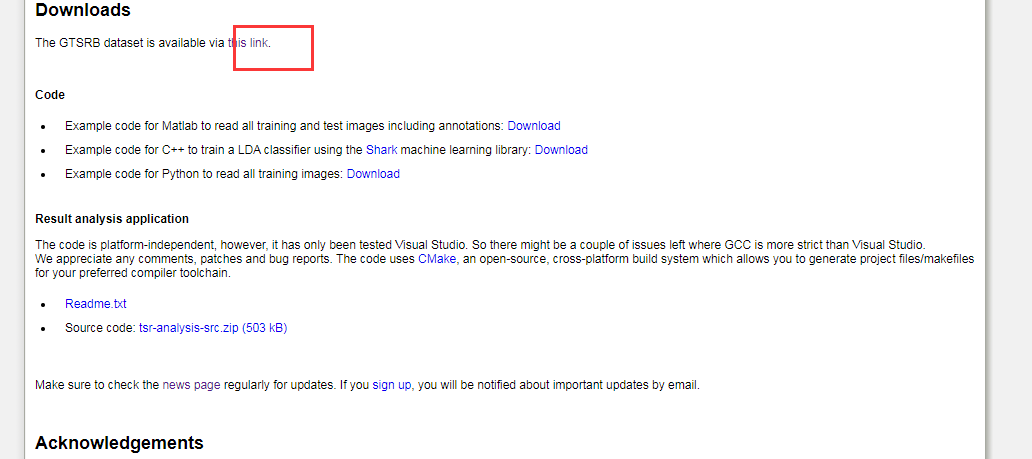这里想要先去吐槽一下，找了好几遍才找到了数据集的下载链接，这也太不起眼了吧，我红框标出来了。

点击跳转到了下载页面后就可以进行下载了，下面是需要下载的数据集：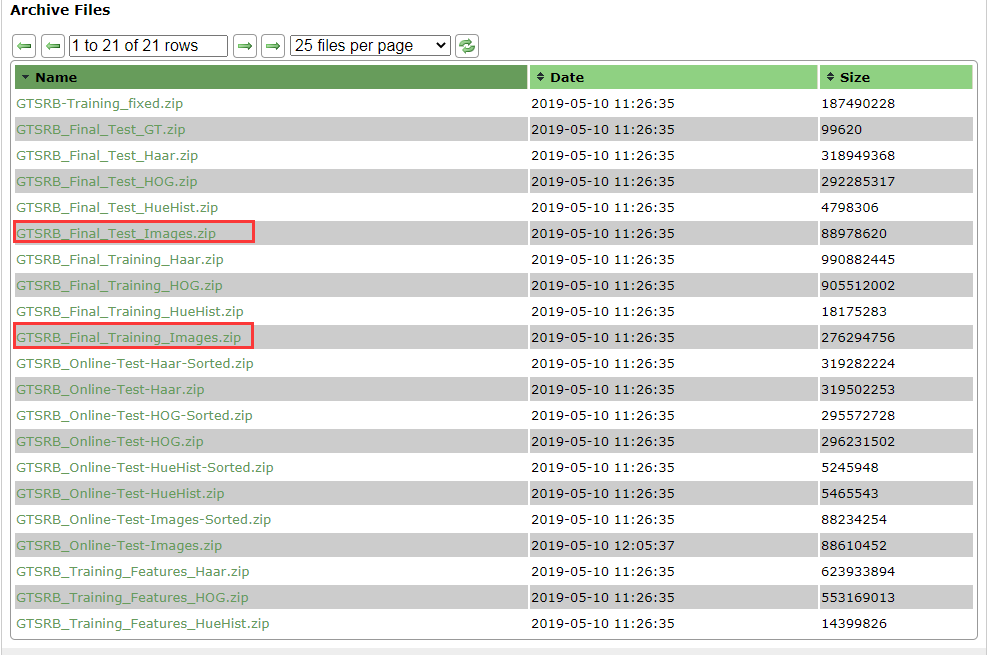当然了，如果不在乎的话也是可以直接全部下载的。

下载后直接解压缩会得到如下文件结构：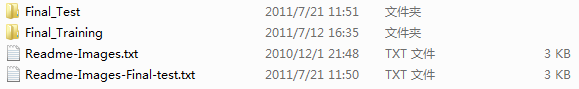我们简单看下测试数据集：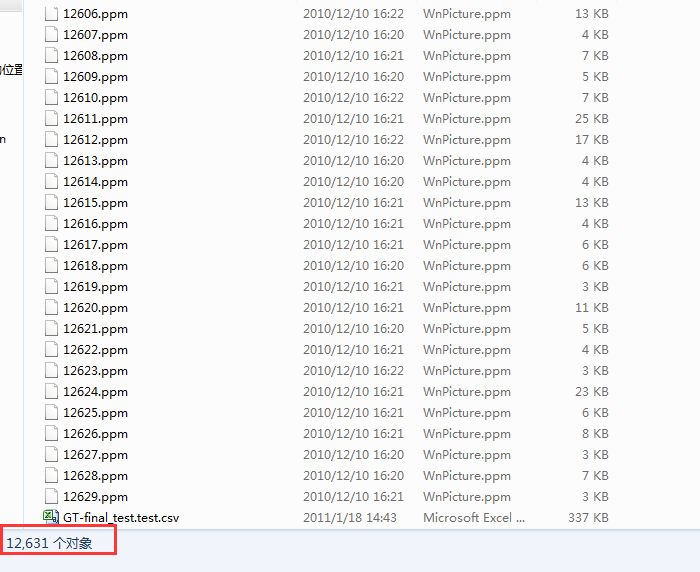可以看到：一共有12630张测试图像，除此之外还有一个csv文件，存放没一张图片的ROI数据，训练集也是同样的组成，接下来我们直接来进行数据解析处理，代码实现如下所示：

#!usr/bin/env python
#encoding:utf-8
from __future__ import division

'''
__Author__:沂水寒城
功能： GTSRB数据集解析处理
'''

import os
import PIL
import numpy as np
import pandas as pd
import matplotlib.pyplot as plt

'''
训练数据集转化处理
'''
for label in labelDir:
oneSaveDir=saveDir+label+'/'
if not os.path.exists(oneSaveDir):
os.makedirs(oneSaveDir)
for one_file in os.listdir(oneDir):
if one_file.endswith(".csv"):
csvFile=os.path.join(oneDir,one_file)
csv_data_array=np.array(csv_data)
for i in range(csv_data_array.shape):
csv_data_list=np.array(csv_data)[i,:].tolist().split(";")
one_ppm=os.path.join(oneDir,csv_data_list)
img=PIL.Image.open(one_ppm)
box=[int(csv_data_list),int(csv_data_list),int(csv_data_list),int(csv_data_list)]
img=img.crop(box)
one_save_path=oneSaveDir+str(len(os.listdir(oneSaveDir))+1)+'.png'
img.save(one_save_path)

'''
测试数据集转化处理
'''
if not os.path.exists(saveDir):
os.makedirs(saveDir)
for one_file in file_list:
if one_file.endswith(".csv"):
csv_data_array=np.array(csv_data)
for i in range(csv_data_array.shape):
csv_data_list=np.array(csv_data)[i,:].tolist().split(";")
img=PIL.Image.open(one_ppm)
box=[int(csv_data_list),int(csv_data_list),int(csv_data_list),int(csv_data_list)]
img=img.crop(box)
one_save_path=saveDir+str(len(os.listdir(saveDir))+1)+'.png'
img.save(one_save_path)

if __name__ == "__main__":

执行，等待一会后，看下处理好的数据集：
首先是【训练集】：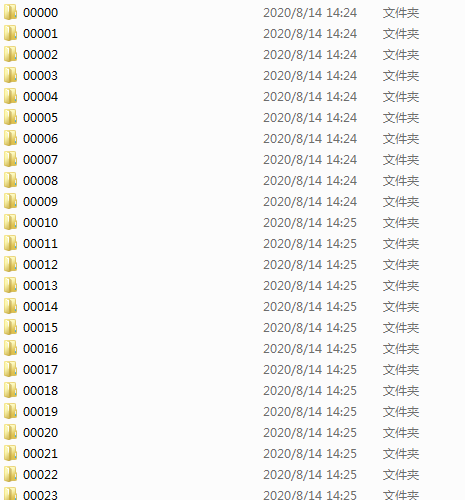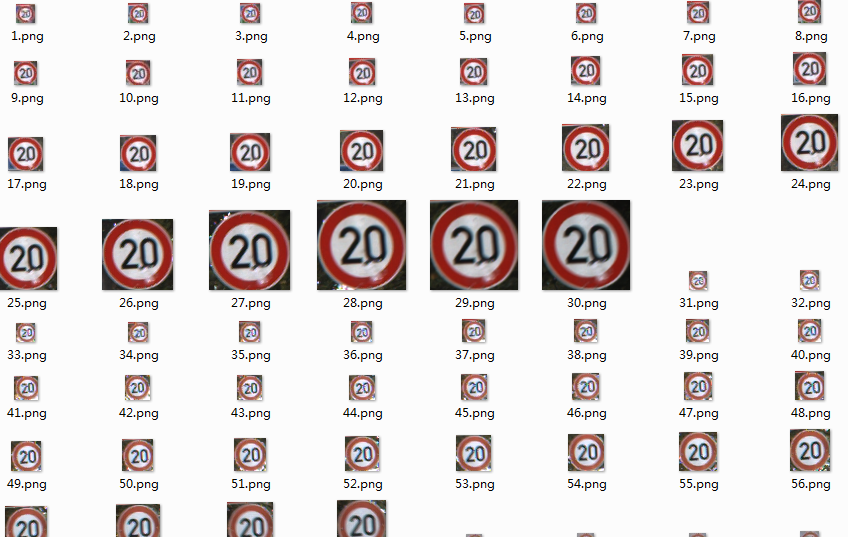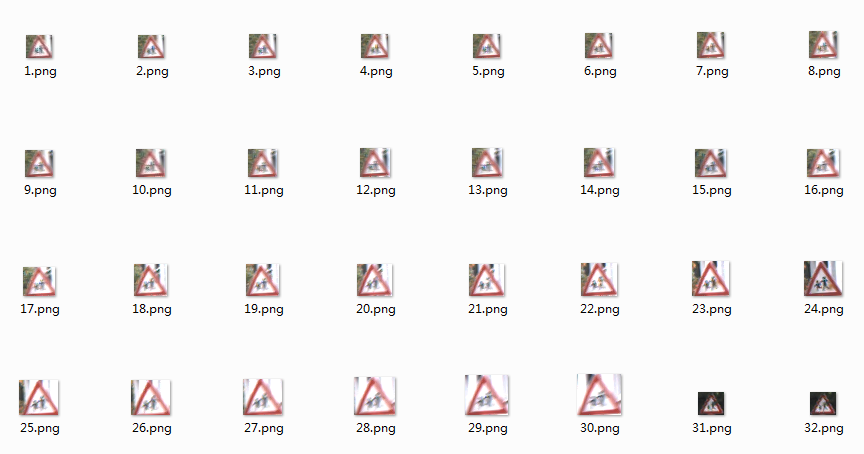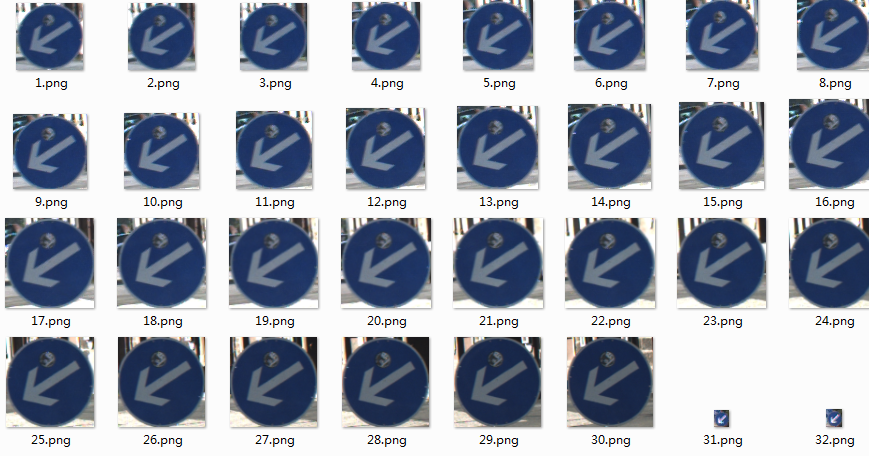接下来是【测试集】：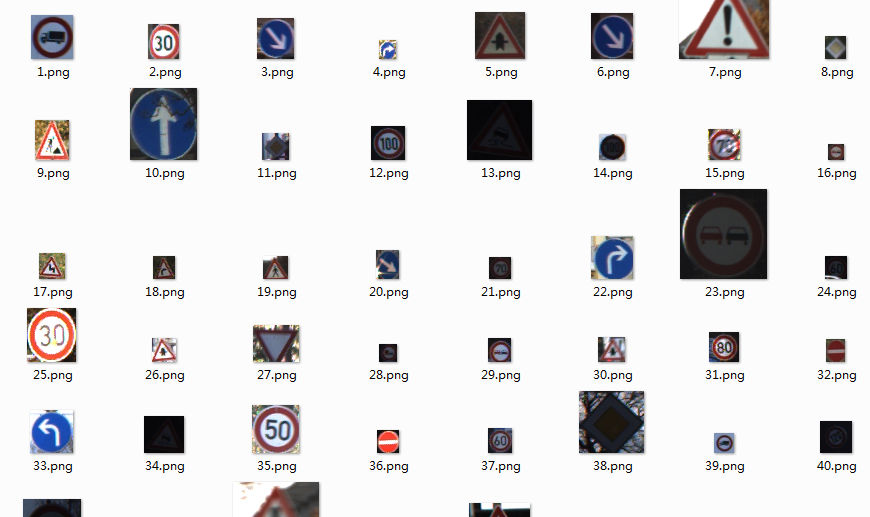完成上述的数据解析存储处理之后就可以进行后续的建模分析了。

展开全文•文档
• 行业资料-交通装置-一种交通标牌的内支撑杆.zip
•文档
• 基于改进卷积神经网络的交通标志牌识别研究.pdf...